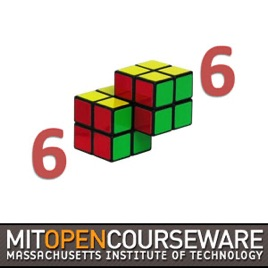47 afleveringen

Lecture videos from 6.006 Introduction to Algorithms, taught by Erik Demaine and Srini Devadas. The course is divided into eight units: introduction, sorting and trees, hashing, numerics, graphs, shortest paths, dynamic programming, and advanced topics.# Introduction to Algorithms (2011) MIT

• Technologie

Lecture videos from 6.006 Introduction to Algorithms, taught by Erik Demaine and Srini Devadas. The course is divided into eight units: introduction, sorting and trees, hashing, numerics, graphs, shortest paths, dynamic programming, and advanced topics.

• video
Lecture 1: Algorithmic Thinking, Peak Finding

## Lecture 1: Algorithmic Thinking, Peak Finding

Overview of course content, including an motivating problem for each of the modules. The lecture then covers 1-D and 2-D peak finding, using this problem to point out some issues involved in designing efficient algorithms.

• 53 min.
• video
Recitation 1: Asymptotic Complexity, Peak Finding

## Recitation 1: Asymptotic Complexity, Peak Finding

This recitation covers asymptotic complexity, recurrences, and peak finding.

• 53 min.
• video
Lecture 2: Models of Computation, Document Distance

## Lecture 2: Models of Computation, Document Distance

This lecture describes an algorithm as a computational procedure to solve a problem, covers the random access machine and pointer models of computation, and introduces the document distance problem.

• 48 min.
• video
Recitation 2: Python Cost Model, Document Distance

## Recitation 2: Python Cost Model, Document Distance

This recitation covers the Python cost model and looks at the code for document distance, including main and most functions except count_frequency.

• 52 min.
• video
Lecture 3: Insertion Sort, Merge Sort

## Lecture 3: Insertion Sort, Merge Sort

Sorting is introduced, and motivated by problems that become easier once the inputs are sorted. The lecture covers insertion sort, then discusses merge sort and analyzes its running time using a recursion tree.

• 51 min.
• video
Recitation 3: Document Distance, Insertion and Merge Sort

## Recitation 3: Document Distance, Insertion and Merge Sort

This recitation continues to look at versions of the document distance code, and briefly discusses insertion and merge sort.

• 54 min.